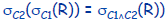# Intro to Database Systems - Part 4 & 5 : Relational Algebra

Let’s start by understanding what relational algebra is:

• it is a query language (not a programming language)
• takes instances of relations as input (basically rows from a table, also called tuples)
• yields instances of relations as output
• uses operators to perform queries (unary - applied on a single relation, or binary - applied on two relations).
• its role? define database operations in terms of algebraic expressions

A query language allows manipulation and retrieval of data from a database. It is useful to explain how a SQL query is executed internally.

Understanding relational algebra is basically understanding SQL. Some basic operations from relational algebra are:

• Select (σ)
• Project (∏)
• Rename (ρ)
• Union (∪)
• Intersection (∩)
• Set difference (-)
• Cross product (X) - also called Cartesian product or Cross join
• Condition join - also called Theta-join
• Equi-join

The select operation is an unary operation noted σc( R ). It returns a subset of rows from the input relation R, satisfying condition C. This constraint is expressed as the predicate. The predicate is a boolean expression whose operators are:

• logical connectives : and, or, not
• arithmetic comparisons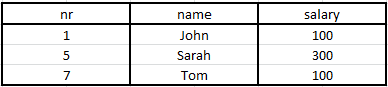Considering a table of employees E, this is an example of the select operation in SQL:

• example 1 : SELECT * FROM E where salary < 200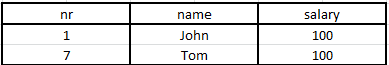• example 2 : SELECT * FROM E where salary < 200 and nr >= 7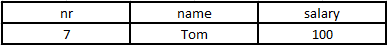Under the relation algebra form, we’ll have:

• σ(salary < 200)(E)
• σ(salary < 200 and nr >= 7)(E)

It is important to notice that:

• the condition C is related to the attributes of relation R
• there are no duplicates in the output
• it’s an easy procedure : for each row, check if condition C is satisfied. If that’s the case, add that row to the result relation.

The project operation is an unary operation noted ∏L ( R ), where L is a list of attributes (A1, A2, A3, …, An) and R is the input relation. It extracts a subset of columns (the attributes) in a relation and discards the rest. If the user is interested in selecting the values of a few attributes, rather than selecting all attributes of the relation/table, one should go for the project operation.

This is an example of the project operation in SQL:

• SELECT salary FROM E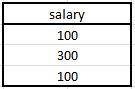• SELECT nr, salary FROM E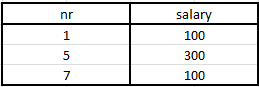Under the relational algebra form, we’ll have:

• ∏(salary)(E)
• ∏(nr, salary)(E)

Note that again, the process is simple:

• extract the columns
• eliminate duplicated rows (NOTE: real systems don’t eliminate duplicated, unless explicitly asked. This operation is very costly.)

We can also use operator composition: embed the result of one operation as an input to another relation. Example:

• in SQL we’ll have : SELECT nr, salary FROM E where salary < 200 and nr >= 7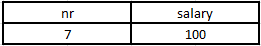• in relational algebra we’ll have : ∏(nr, salary)(σ(salary < 200 and nr >= 7)(E))

The rename operator is a unary operator noted ρ( X(x1,x2,…,xn), Y(y1, y2, …, yn)) where X is identical to the initial relation Y, but with a new relation name X and new attribute names (x1 to xn). Example:

• in SQL we’ll have : SELECT nr AS ‘Employee_Number’, salary AS ‘Monthly_Salary’ FROM E
• in relational algebra we’ll have : ρ( EMPLOYEE(Employee_Number, Monthly_Salary), E(nr, salary))

The union operator takes as input two relations R and S.

• it joins or includes all tuples (rows) that are in R or in S
• duplicate tuples (rows) are eliminated
• both relations should have the same number of attributes (columns)
• attribute domains must be compatible

As an example,

• in SQL we’ll have : SELECT * FROM R UNION SELECT * FROM S
• in relational algebra we’ll have : R ∪ S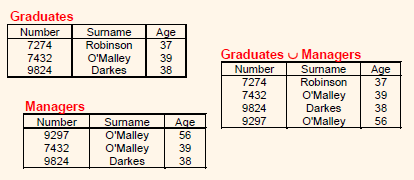The intersection operator is applied when only rows shared by both A and B are included in the result. It has the same preconditions as the union operator (no duplicates, same number of attributes, domain compatibility).

• in SQL we’ll have: SELECT * FROM A INTERSECT * FROM B
• more particularly, we can also an intersection of a specific attribute : **SELECT Surname FROM Graduates INTERSECT Surname FROM Managers
• in relational algebra, the general case is represented by : A ∩ B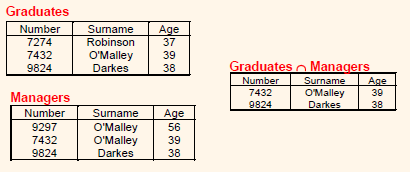The set difference operator between two relations A - B results in a relation containing rows that are in A but not in B.

• in SQL we’ll have: SELECT * FROM A MINUS SELECT * FROM B
• again, we can have a particular case where we consider only certain attributes (columns): SELECT Surname FROM Graduates MINUS Surname FROM Managers
• in relational algebra, the general case is represented by : A - B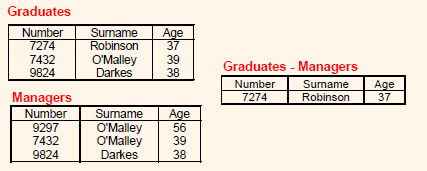The cross product operator between two relations A X B is the set of pairs that can be formed by pairing each row of A with each row of B.

• in SQL we’ll have: SELECT * FROM A, B
• in relational algebra we’ll have: A X B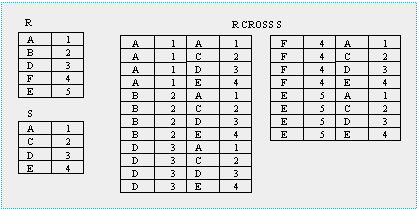If it happens that attributes have the same name in the resulting relation, we should prefix the similar attributes with the initial relation name (example : id is an attribute of relation school, but also of relation library. In the resulting relation, there should be an attribute for school id and another one for library id).

An easy way to implement the cross product operator is a nested loop. For each row from table A, pair it with a row from table B.

The condition join is just a cross product satisfying a condition. We apply the nested loop method in order to create the pairs, but only in the case where the condition is satisfied. It’s the selection operation applied to a cross product.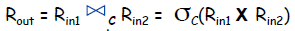In SQL we’ll have : SELECT * FROM Employee, Department WHERE dept = depNr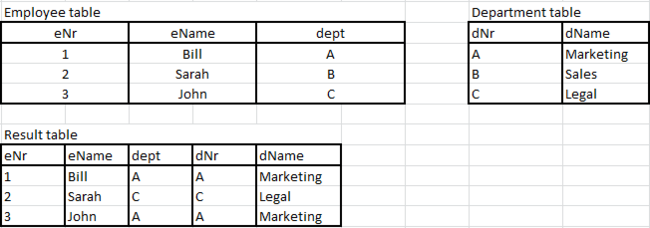The equi-join is simply a condition join where the condition is an equality.

The natural-join is an equi-join if values from columns with the same name are equal. If relation A has attribute (column) studID and relation B has attribute studID, rows will be paired only if their value are identical (relation A has a student with studID 99 and relation B has a student with stutID 99). In other words, we need 2 conditions to apply the paring:

• column name is the same in both relations
• row value under that column is the same in both relations.

As a conclusion, here are some properties of relational algebra:

• Commutativity between project and select, if the condition C considers attributes of the subset L.
• Commutativity between cross product inputs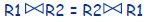• Associativity between cross product inputs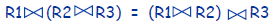• Idempotence of project operator, if L2 is a subset of L1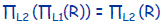• Idempotence of select operator